# EVALUATING FUND PERFORMANCE WITHIN THE STOCHASTIC DISCOUNT FACTOR FRAMEWORK (Finance)

## Abstract

The stochastic discount factor (SDF) approach to fund performance is a recent innovation in the fund performance literature (Knez, 1996). A number of recent studies have used the stochastic discount factor approach to evaluate the performance of managed funds. In this paper, I present an overview of the use of the stochastic discount approach to evaluate the unconditional and conditional performance of the fund. I also discuss estimation issues and provide a brief survey of empirical evidence.

## Introduction

Evaluating the performance of a managed fund has a long and illustrious history since the seminal studies of Sharpe (1966) and Jensen (1968). There have been numerous performance measures developed and used in the literature during the past thirty-five years. A partial list of these measures includes the unconditional Jensen measure (extended by Connor and Korajczyk, 1986), the selectivity and market timing measures of Treynor and Mazuy (1966) and Hendriksson and Merton (1981), the period weighting measure of Grinblatt and Titman (1989), the portfolio weight measures of Cornell (1979), Grinblatt and Titman (1993), Daniel et al. (1997), and Ferson and Khang (2002), and the conditional performance measure of Ferson and Schadt (1996). A recent innovation in the fund performance literature has been the development of performance measures within the stochastic discount factor (SDF) approach. A major attraction of the stochastic discount factor approach is that most asset pricing models can be written as a candidate model of the SDF.

Knez (1996) present a general framework to evaluate fund performance within the SDF approach1.Knez also explore the minimal conditions under which valid performance measures exist. The SDF approach to fund performance has been used and developed in a number of recent studies such as Dahlquist and Soderlind (1999), Farnsworth et al. (2002), Ferson et al. (2003), Fletcher and Forbes (2004), and Lynch et al. (2004) among others. The SDF performance measures of Knez have also been used to examine the profitability of momentum trading strategies (Ahn et al., 2003c) and seasoned equity offerings (Ahn et al., 2003b).

In this paper, I present an overview of the SDF approach to fund performance and discuss a number of estimation issues in using the SDF approach. The paper is organized as follows. Section 12.2 provides an overview of the SDF approach to fund performance. Section 12.3 discusses estimation issues. Section 12.4 presents a summary of empirical findings. The final section concludes.

## Evaluating Performance

Ross (1978), Harrison and Kreps (1979), and Hansen and Richard (1987), among others, show that if the law of one price holds in financial markets, then there exists a stochastic discount factor mt such that: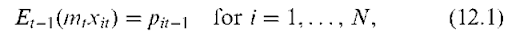where xit is the payoff of asset i at time t, pit-1 is the price of asset i at time t— 1, N is the number of primitive assets, and Et—1 is the expected value conditional on the information available to investors at time t— 1. Where the payoff of asset i is equal to the gross return of asset i, the payoff equals 1. Where the payoff of asset i is an excess return, the price equals 0. Equation (12.1) states that the expected value of the risk-adjusted payoff of asset i at time t conditional on information available at time t— 1 has a price equal to pit—1. Under the assumption of no arbitrage, mt will be positive in every state of nature (Cochrane, 2001). In complete markets, mt will be unique.

### Equation (12.1) can be written in terms of excess returns as: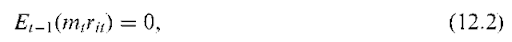where rit is the excess return of asset i in period t. Where there is no conditioning information, Equation (12.2) implies that: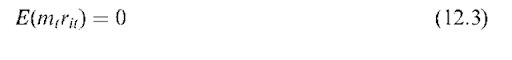### Equation (12.3) can be rearranged using the definition of covariance as: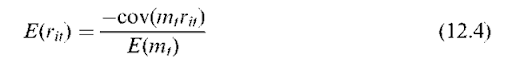Equation (12.4) states that the expected excess return of asset i depends upon the covariance between the SDF and excess return (cov(mtrit)). The cov(mtrit) captures the risk adjustment implied by the SDF model. Assets with a negative covariance with the SDF have higher expected excess returns.

Knez (1996) present a general framework to evaluate fund performance using a candidate model of the SDF. Define yt as a candidate model of the SDF. Knez (1996) show that the unconditional performance of the fund can be written as: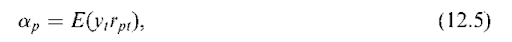where ap is the performance of the fund and rpt is the excess return of the fund in period t. The performance of the fund (ap) measures the difference between the expected risk-adjusted excess return of the fund and its price (0). If performance is positive (negative), the fund offers a higher (lower) risk-adjusted excess returns than expected, which signifies superior (inferior) performance.

### The conditional performance of the fund is given by: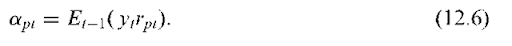The conditional performance of the fund apt measures the difference between the expected risk-adjusted excess return of the fund at time t conditional on information available at time t— 1 minus its price. The conditional performance of the fund varies over time as a function of conditioning information.

What is the goal of a performance measure? Knez (1996) point out that a performance measure seeks to measure the value added by a professional portfolio manager. Does the portfolio manager enlarge the investment opportunity set of investors? This question can be addressed by using unconditional performance measures or conditional performance measures. An unconditional framework assumes that the investment opportunity set of uninformed investors is spanned by passive trading strategies in the N primitive assets. A conditional framework allows for uninformed investors to follow dynamic trading strategies in the N primitive assets based on publicly available information.

Grinblatt and Titman (1989) and Knez (1996) point out that a good performance measure should have two characteristics. First, any trading strategy that can be achieved by uninformed investors should be given zero performance. Second, trading strategies followed by portfolio managers with superior information should be given positive performance. If a performance measure satisfies the first characteristic, it is defined as an admissible performance measure.Knez show that the first characteristic is met if there exists a SDF that correctly prices the set of primitive assets that uninformed investors can trade in. This result implies that an admissible performance measure is equivalent to using a valid SDF model. Such SDFs will exist if the law of one price (LOP) holds in financial markets (Knez, 1996). Admissible performance measures can also be consistent with no arbitrage (NA) opportunities in financial markets.

The use of the conditional performance framework provides a greater challenge to the portfolio manager because valid SDF models will be able to price not only the N primitive assets but also dynamic trading strategies in the primitive assets. Portfolio managers who trade on the use of public information will not be rewarded superior performance within the conditional framework. In contrast, the portfolio manager can be rewarded superior performance by trading on public information in an unconditional framework (see Ferson and Schadt, 1996; Ferson and Khang, 2002; Ferson, 2003, for further discussion).

The sensitivity of fund performance to the SDF model used is related to the literature that shows the sensitivity of the Jensen (1968) performance measure to the benchmark portfolio used (Roll, 1978; Lehmann and Modest, 1987). Much of the debate about how sensitive the Jensen performance of the fund is to the benchmark portfolio stems from the use of inappropriate benchmarks i.e. inadmissible performance measures. The analysis in Knez (1996) is more serious in that fund performance is sensitive to the SDF model used even for admissible measures.

Ahn et al. (2003a) build on the earlier work of Chen and Knez (1996) to derive the upper and lower performance bounds for a given fund under the conditions that the SDF model prices the primitive assets and satisfies the no arbitrage condition. Ahn et al. show that for a given set of primitive assets, all admissible performance measures for a given fund will lie within these bounds. The performance of the fund will only be unambiguous when the lower bound lies above zero (positive performance) or the upper bound lies below zero (negative performance). When the bounds straddle zero, admissible performance measures can give the same fund positive or negative performance.

## Estimation Issues

The estimation of fund performance, within the SDF framework, is conducted using Generalized Method of Moments2 (GMM) (Hansen, 1982). One approach is to use a two-step approach. First, the coefficients in the candidate SDF model are estimated. Second, the performance of the fund is estimated as in Equation (12.5) by multiplying the fund’s excess return by the SDF model and taking the average. An alternative approach is to estimate the coefficients in the SDF model and the performance measure jointly. Farnsworth et al. (2002) advocate this approach, as it is more efficient than a two-step approach. I will discuss the estimation of the unconditional performance measure first, and then move on to the conditional measures.

### Define the following set of residuals for a given candidate model of the stochastic discount factor yt: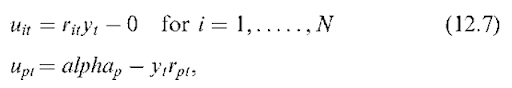where alphap is the unconditional performance of the fund. The sample mean of the residuals are the moment conditions in GMM estimation. The first N moment conditions identify the K parameters in the SDF model yt. These moment conditions are also the pricing errors of the N primitive assets. The last moment condition identifies the performance of the fund. There are N + 1 moment conditions and K +1 parameters in the system of Equations in (12.7). When N + 1 = K + 1, the system of equations is exactly identified and there are no over identifying restrictions. Under the null hypothesis of no abnormal performance alphap should be equal to zero.

Define g as the (N + 1)*1 vector of the sample mean of residuals (moment conditions). GMM estimates the K + 1 parameters to minimize the quadratic form g’Wg, where W is an (N + 1)*(N + 1) arbitrary weighting matrix. Hansen (1982) shows that the estimates have an asymptotic normal distribution for any arbitrary weighting matrix. Hansen also shows that the most efficient weighting matrix is S~1, where S is the covariance matrix of the moment conditions. The advantage of the GMM approach is that it is valid under general distributional assumptions and we can incorporate the effects of serial correlation and heteroskedasticity.

When we estimate the performance of more than one fund, the number of moment conditions increase sharply. However, Farnsworth et al. (2002) show that the estimated performance and standard error of the fund is invariant to the number of funds used in the estimation. This result implies there are no biases in the performance (or standard error) for a given fund by excluding other funds from the estimation. This finding is encouraging given the number of funds used in empirical studies. Ferson et al. (2003) generalize this result to conditional time-varying performance measures.

We can include additional moment conditions to the system of Equations in (12.7) to incorporate additional restrictions implied by SDF models. Dahlquist and Soderlind (1999) and Farnsworth et al. (2002) show that it is important to add a moment condition for the gross risk-free return. The expected value of the SDF should be just below one (see Cochrane, 2001). By including this moment condition, the expected value of the SDF model has more sensible values. Farnsworth et al. also point out that for linear factor models of the SDF, where the factors are portfolio returns, it is important to impose the restriction that the model correctly prices the factors.

Two issues arise using GMM to estimate the unconditional performance of the fund. First, the researcher must choose the set of N primitive assets. This set should capture the investment opportunity set that investors can trade in. The number of primitive assets should also be small. Cochrane (2001) recommends that the number of moment conditions should be at most one-tenth of the number of time-series observations because the estimate of S can become unstable and near singular, when the number of moment conditions is too high. Different sets of primitive assets have been used in the literature. Chen and Knez (1996), Dahl-quist and Soderlind (1999), and Ahn et al. (2003a) all use industry portfolios in their set of primitive assets. Dahlquist and Soderlind also add a short-term Treasury Bill to the set of primitive assets. Farnsworth et al. (2002) use two bond portfolios, one-month Treasury Bill, and six stock portfolios that capture small cap/large cap, value/growth, and momentum/contrarian investment strategies. One issue that arises is whether fund performance is sensitive to the choice of the primitive assets. Ahn et al. (2003d) propose further discussion of this issue in an asset pricing context, and propose a novel approach to form the set of primitive assets.

Second, what weighting matrix should be used in estimating the system of Equations in (12.7). The issue of the weighting matrix can be important whenever the number of parameters is less than the number of moment conditions. A major problem in using the optimal weighting matrix S_1 is that in small sample sizes the optimal weighting matrix can perform poorly (Lettau and Ludvigson, 2001). The optimal weighting matrix can suffer from two other problems whenever we want to consider how well different models of yt price the N primitive assets. First, the optimal weighting matrix is different across each model, and so cannot be used to compare the pricing performance of different models. Using the optimal weighting matrix, improvements in model performance can come from lower pricing errors and a more volatile weighting matrix. Second, the optimal weighting matrix evaluates the ability of SDF models to price the primitive assets in terms of how well the model prices portfolios of large, long, and short positions (Cochrane, 2001). Cochrane and Chretien and Cliff (2001) show that the optimal weighting matrix estimates the parameters in the SDF to price the sample global minimum variance portfolio as well as possible.

The two most popular alternative weighting matrixes that can be used to evaluate fund performance are the ones proposed in the asset pricing literature. Cochrane (1996) advocates the use of the identity weighting matrix and Hansen and Jagannathan (1997) advocate the use of the inverse of the second moment matrix of asset payoffs. Since the same weighting matrix is used across models, we can evaluate how well different models price the primitive assets. The identity weighting matrix places an equal weight on each moment condition. In terms of evaluating asset pricing models, the identity matrix estimates the parameters to minimize the sum of squared pricing errors. This approach is most useful whenever the researcher wants to examine how well models price a given set of assets rather than complex long/short portfolios of assets. However, the use of the identity weighting matrix can lead to more volatile estimates of the parameters (Hodrick and Zhang, 2001).

The use of the Hansen and Jagannathan (1997) weighting matrix in the GMM minimization g’Wg is equal to the squared Hansen and Jagannathan distance measure under the LOP assumption. The distance measure captures the smallest distance between a given candidate model of the SDF and the true set of discount factors that price the primitive assets. The distance measure is also the most mispriced portfolio of the primitive assets with unit norm. Asset pricing models that are more able to price the primitive assets should have lower distance measures. Given the choice of weighting matrixes available, an interesting study would be to explore whether fund performance is sensitive to different weighting matrixes.

The GMM framework can be extended to estimate the conditional performance measures. To estimate the average conditional performance as in Farnsworth et al. (2002), we can add additional moment conditions to capture the unconditional implications of conditioning information as in Cochrane (1996, 2001). Define z1t_1 as the value of the lth information variable at time t— 1. Cochrane shows that if we multiply both sides of Equation (12.2) by zlt—1, and take unconditional expectations that: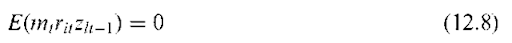The payoff ritzit—1 is the payoff of a dynamic trading strategy that has a zero price. Cochrane shows that this approach is sufficient to test all the implications of conditioning information. The approach has the attractive feature that it is still valid even if the researcher uses smaller information set than observed by investors. Using less information variables than observed by investors reduces the power of the tests (Cochrane, 1996).

For every information variable zlt— 1 used by the researcher, there are N additional moment conditions. The restrictions from Equation (12.8) imply the following residuals: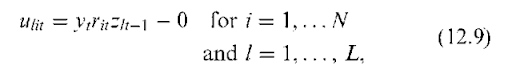where L is the number of common information variables. We can estimate the average conditional performance as in Farnsworth et al. (2002) by adding the L*N extra moment conditions from Equation (12.9) to the system of Equations in (12.7). In this situation, the alphap coefficient is the average conditional performance of the fund.

We can estimate time-varying conditional performance by assuming that the performance of the fund is a linear function of the common information variables3 as in Dahlquist and Soderlind (1999) and Lynch et al. (2004). The extra parameters in the conditional performance function can be estimated by adding additional moment conditions to the system of Equations in (12.7) and (12.9). An alternative approach to the linear functional form followed by Ferson et al. (2003) who use a small number of conditioning dummy variables that capture different states of the term structure.

The SDF approach is a very general approach to fund performance and a wide range of alternative models can be used. The models include different versions of the consumption asset pricing model or production based asset pricing models can be used. The most popular models used in the evaluation of fund performance are linear factor models such as the capital asset pricing model (CAPM) or arbitrage pricing theory (APT).

Models such as the CAPM and APT imply a linear model of the SDF (see Cochrane, 2001; Ferson, 2003, for a review). In the unconditional versions of the models where the coefficients in the model are assumed constant through time, the SDF can be written as: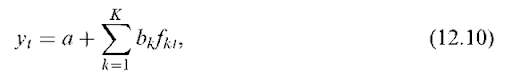where fkt is the value of factor k in period t, a is the constant in the linear model, bk is the slope coefficient relative to the kth factor (for k = 1,…, K), and K are the number of factors in the model. The slope coefficients bk capture the importance of each factor in the SDF model. The factors fkt can be excess returns on portfolios or zero-cost portfolios, or aggregate macroeconomic variables, or state variables that predict changes in the investment opportunity set. Unconditional models assume that the betas and factor risk premiums are constant through time. Conditional versions of the models can be used by assuming that the coefficients in the model are a linear function of the common information variables as in Cochrane (1996) and Lettau and Ludvigson (2001) among others4.

An alternative approach is not to rely on an asset pricing model at all, and use nonparametric performance measures such as in Chen and Knez (1996) or the numeraire portfolio of Long (1990). The Chen and Knez measures rely on less restrictive assumptions than an asset pricing model such as the LOP. The SDF used by Chen and Knez under the LOP builds on the earlier work of Han-sen and Jagannathan (1991). Hansen and Jagan-nathan show that there exists under the LOP, an unique SDF that correctly prices the primitive assets and is also a portfolio payoff. This unique SDF is a linear function of the N primitive assets. This approach can be modified to impose the no arbitrage condition.

## Empirical Evidence

There have been numerous empirical studies evaluating fund performance during the past three decades. The number of studies using the SDF is small. Chen and Knez (1996) use their LOP and NA measures to evaluate the performance of a sample of 68 U.S. mutual funds between 1968 and 1989. Chen and Knez find little support of superior performance by funds and the average fund performance is —0.09 percent using the unconditional LOP and NA measures. Dahlquist and Soderlind (1999) use the Chen and Knez measures to evaluate the small sample properties of the performance tests and the performance of Swedish mutual funds using weekly data. Dahlquist and Soderlind find that the asymptotic tests can perform poorly in small samples and reject the null hypothesis of zero performance too often when there is no abnormal performance. In addition, the power of the tests can be low because detecting true superior performance requires a large abnormal return and a long sample period. Dahlquist and Soderlind find that the average Swedish mutual fund provides small positive performance, but is not statistically significant.

Ahn et al. (2003a) estimate the upper and lower performance bounds for 320 U.S. mutual funds between 1984 and 1997 using the set of admissible performance measures under the no arbitrage condition. Ahn et al. find that for 80 percent of the funds, the performance is sensitive to the SDF model used. There are valid SDF models that assign the same fund positive or negative performance. Where the valid SDF models agree on the performance of the fund, the results support the existence of inferior performance.

Ahn et al. (2003a) also use the performance bounds to conduct diagnostic tests on different performance measures used in the academic literature. Ahn et al. consider the Jensen (1968) and Ferson and Schadt (1996) measures using the CAPM, the three-factor Fama and French (1993) model, and the four-factor model used in Ferson and Schadt (1996), two consumption-based models using the standard time-separable power utility function and an external habit function, and the Chen and Knez (1996) LOP and NA measures. Among the linear factor models, Ahn et al. find that the conditional Fama and French model have the smallest proportion of funds that have performance measures that lie outside the bounds. The two consumption models perform poorly with a substantial number of funds having performance measures outside the bounds. The performance of the funds using the Chen and Knez LOP measure falls out with the bounds in only 0.62 percent of cases.

Farnsworth et al. (2002) provide a comprehensive examination of fund performance across a wide class of SDF models. The models used are five linear factor models (CAPM, Fama and French (1993), three-factor APT, three-factor model using traded factors, and four-factor model using macro-economic variables), the Chen and Knez (1996) LOP model, the numeraire portfolio of Long (1990), and the Bakashi and Chen (1998) model. Conditional and unconditional versions of the models are used. Farnsworth et al. examine the performance of the different models to price the primitive assets using the Hansen and Jagannathan (1997) distance measure, and also consider how well the models capture the time-series predictability of the pricing errors of the primitive assets. Farnsworth et al. find that conditional models are better able to capture the time-series predictability in pricing errors and have lower Hansen and Jagan-nathan distance measures in most cases when dynamic trading strategies of the primitive assets are included. However, this improved performance of the conditional model comes at the expense of higher unconditional Hansen and Jagannathan distance measure.

Farnsworth et al. (2002) use hypothetical trading strategies to examine whether the different models assign zero performance for strategies with no skill, and if the models can detect significant superior performance. The trading strategies allow for varying levels of stock selection and market timing skill. Farnsworth et al. find that there is a small downward bias in performance for the stock selection strategies with no skill of the order of —0.19 percent for unconditional models and —0.12 percent for conditional models. Most of the models are able to detect significant superior performance for strategies with varying degrees of stock selection and market timing ability. The poorest performing models at detecting superior performance are the numeraire portfolio model of Long (1990) and the four-factor linear model using macroeconomic variables. Performance findings are similar across the remaining models.

When the models are used to evaluate the performance of a sample of U.S. mutual funds, Farnsworth et al. (2002) find that the average fund performance across models is —0.06 percent for unconditional models and —0.09 percent for conditional models. Adding back annual expenses and trading costs, the average mutual fund earns better performance than the hypothetical trading strategies with no skill. There is little evidence of superior performance by U.S. mutual funds. Fletcher and Forbes (2004) also find little evidence of superior performance by U.K. unit trusts using a wide range of SDF models.

Lynch et al. (2004) evaluate the conditional performance of U.S. mutual funds between 1977 and 1993. Lynch et al. use the CAPM, Fama and French (1993), and Carhart (1997) models to evaluate fund performance, and use the dividend yield on the market index as the information variable. The dividend yield is used to track the variation in the business cycle. Lynch et al. finds that conditional performance of funds varies over time. There are also interesting patterns in the conditional performance across different fund investment sectors. The abnormal performance of growth funds rises during booms and falls during downturns. The converse is true of the other investment sectors5.

Ferson et al. (2003) use the SDF approach to evaluate the conditional performance of U.S. government bond mutual funds between 1986 and 2000. The models used are based on continuous-time term structure models. Ferson et al. use reduced form SDF models for the one-factor affine model, the two-factor affine model, the three-factor affine model, and the two-factor Bren-nan and Schwartz (1979) model. The empirical versions of the models include additional time-averaged factors due to using the models over discrete periods of time. This approach has the advantage of dealing with the interim trading bias of Goetzmann et al. (2000) and Ferson and Khang (2002). Ferson et al. (2003) use conditioning dummy variables to estimate fund performance over different states of the term structure.

Ferson et al. (2003) conduct a number of diagnostic tests of the different term structure models. Ferson et al. find that the additional empirical factors play an important role in explaining bond returns. The one-factor affine model has the poorest performance in pricing different bond portfolio strategies. The two-factor models perform better than the one-factor affine model, and the three-factor affine model has the best performance. Ferson et al. find that government bond performance varies across states of the term structure. Although there is little evidence of superior performance, some types of funds perform better in certain states of the term structure. In low-short rates, young funds, low turnover, low loads, low expenses, and low total costs all have significant positive performance.

## Conclusions

The SDF approach to evaluate fund performance is a recent innovation in the fund performance literature. The SDF approach has a number of attractive features in that most asset pricing models imply a candidate model of the SDF and the approach can be applied to conditional performance evaluation. A small number of studies have evaluated fund performance within the SDF approach, and find little support for superior performance. It would be interesting to compare the SDF approach to fund performance to the more traditional Jensen (1968) and Ferson and Schadt (1996) measures based on linear-beta models. There has been a lively debate in the academic literature recently about the relative merits of the two alternative approaches in testing asset pricing models (see Kan and Zhou, 1999; Jagannathan and Wang, 2002). There is also wide scope for using the SDF approach to examine the conditional performance of different types of funds.

Previous post: